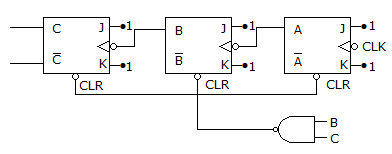# Electronics and Communication Engineering - Digital Electronics - Discussion

34.

The counter in the given figure is[A]. Mod 3 [B]. Mod 6 [C]. Mod 8 [D]. Mod 7

Explanation:

When counter is 110 the counter resets. Hence mod 6.

 Sharon said: (Mar 16, 2015) Expand above expression?

 Vijaya Venkat said: (May 31, 2015) Actually A is LSB and C is MSB. Here clear is active low so that we have to give clr=0. For that B and C should be 1 and 1. Because the output of NAND must 0.

 Deblina Mukherjee said: (Aug 8, 2016) Not getting please elaborate, how 6?

 Rakesh said: (Aug 21, 2016) Of course @Vijaya Venkat, The answer follows like this I think. 000, 001, 010, 011 then counter resets. So the answer must be mod 3.

 Lal said: (Sep 16, 2016) I think the states will be like this, 000 101 011 100 101 110 (but the flipflops would reset at this state) so it is a mod 6 counter.

 Jay Bhatt said: (Aug 18, 2017) Answer should be MOD 7. Because if it starts from 000 (A,B and C respectively) it will go as below, ABC 000 100 010 110 001 101 011 back to 000 Hence it is Mod 7.

 Darshith said: (Dec 4, 2017) I think its Better to Mention Which one is MSB and LSB in the question.

 Vijayalakshmi said: (Oct 20, 2018) JK flip flop state is 2N:1. N=3 flip flop,so mod=2N=2*3=6. Ans:B.

 Bhanu said: (Jan 11, 2019) Mod 3 is correct answer. Because MSB is always at right side in counters topic itself.

 Ashish said: (Jan 22, 2019) It is asynchronous counter with A as LSB (as clk is applied to A only). Hence only up counting or down counting takes place. Mod 6 is correct.

 Pryanka Yadav said: (Jan 10, 2020) Given Mod-6 counter is correct.

 Karthikraj said: (Apr 23, 2021) We get a high o/p at MSB ff is high. To get this B, C should be 1 and A is 0 to clock the middle ff. To get high at o/p is itself a reset state. Hence 110 is a toggle for reset. B=C=0 or B=0 and C=1 or vice versa are not possible. Hence the count is the answer i.e. mod-6.

 Vishnu said: (May 8, 2021) Here is the trick; a(lsb) b c(msb) a and b set to clear So 110==4+2+0=mod-6.